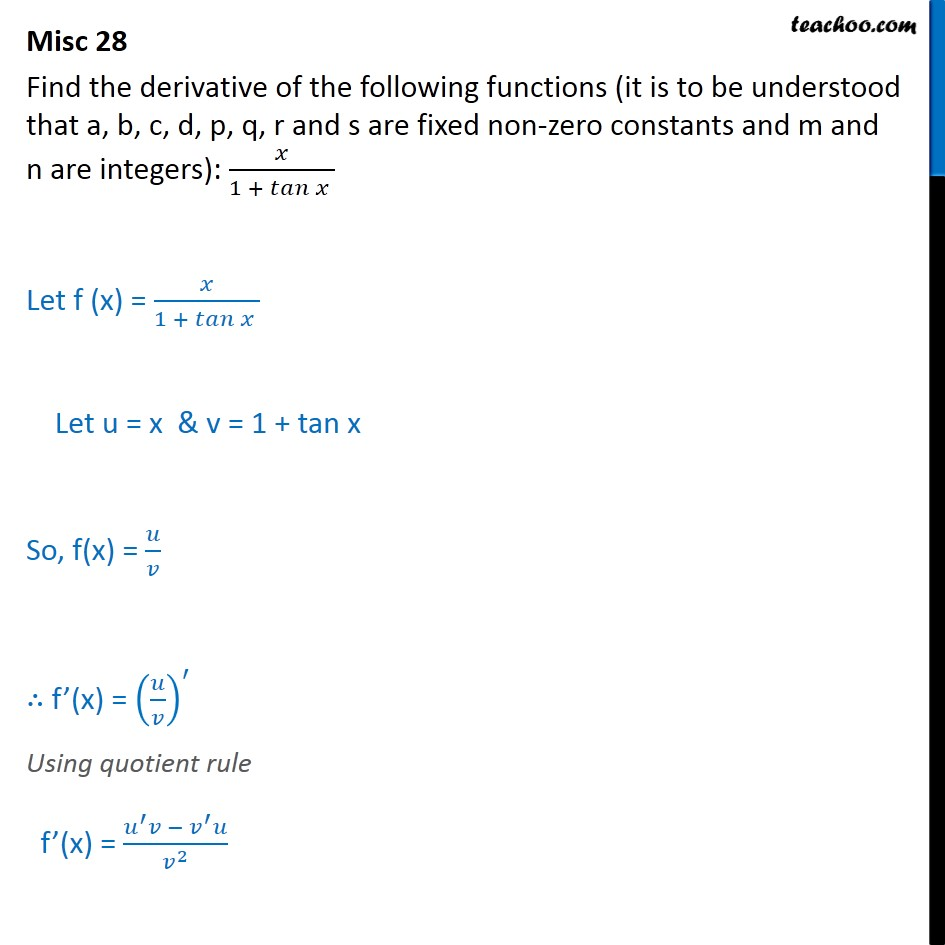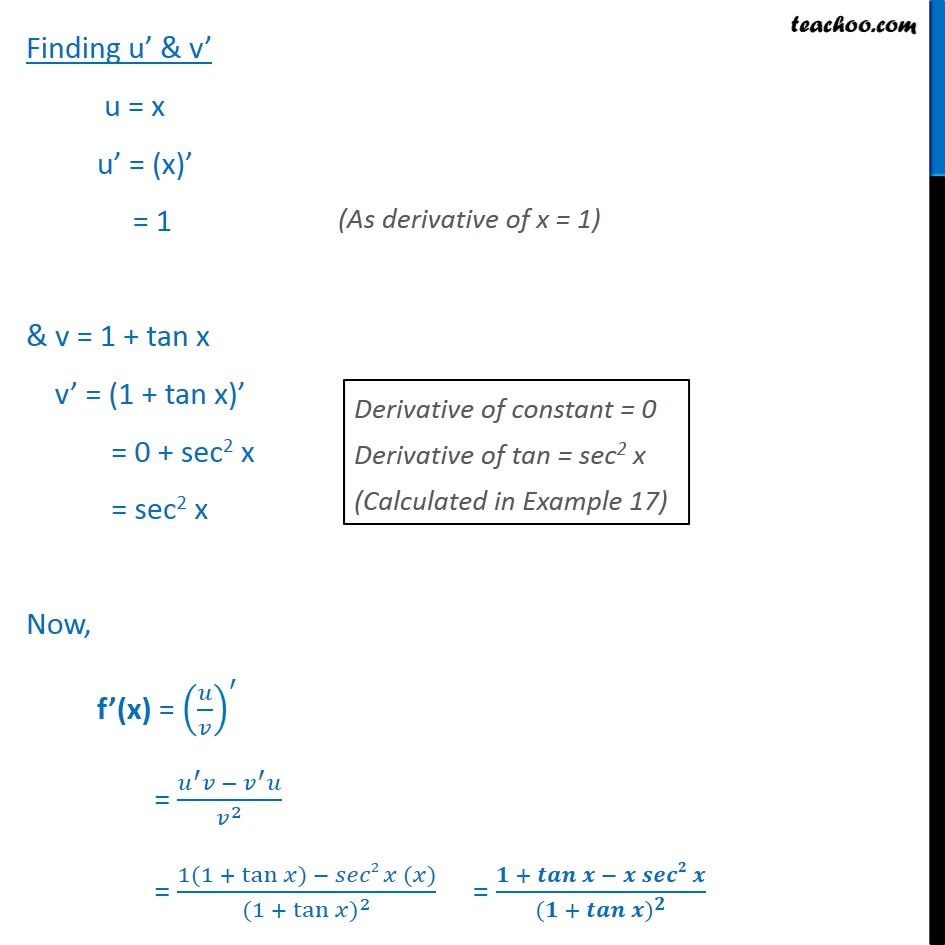1. Class 11
2. Important Question for exams Class 11
3. Chapter 13 Class 11 Limits and Derivatives

Transcript

Misc 28 Find the derivative of the following functions (it is to be understood that a, b, c, d, p, q, r and s are fixed non-zero constants and m and n are integers): 1 + Let f (x) = 1 + Let u = x & v = 1 + tan x So, f(x) = f (x) = Using quotient rule f (x) = 2 Finding u & v u = x u = (x) = 1 & v = 1 + tan x v = (1 + tan x) = 0 + sec2 x = sec2 x Now, f (x) = = 2 = 1(1 + tan ) 2 ( ) (1 + tan ) 2 = + ( + )

Chapter 13 Class 11 Limits and Derivatives

Class 11
Important Question for exams Class 11

About the AuthorDavneet Singh
Davneet Singh is a graduate from Indian Institute of Technology, Kanpur. He has been teaching from the past 9 years. He provides courses for Maths and Science at Teachoo.